## Figures index

#### C. Nwoye, E. C. Chinwuko, P. C. Nwosu, N. E. Idenyi

International Journal of Materials Lifetime. 2015, 2(1), 13-21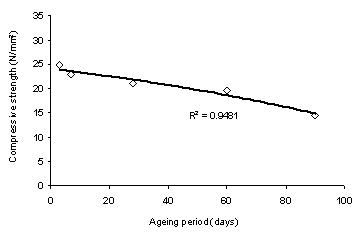• Figure 1. The coefficient of determination between the compressive strength of concrete and its ageing period as obtained from experiment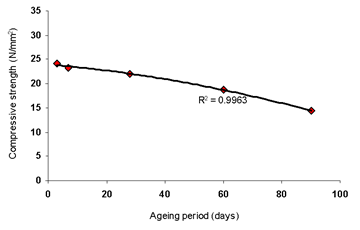• Figure 2. The coefficient of determination between the compressive strength of concrete and its ageing period as predicted by derived model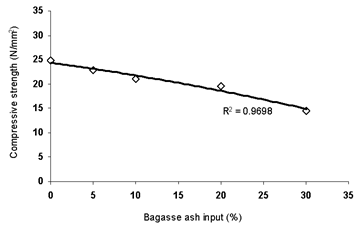• Figure 3. The coefficient of determination between the compressive strength of concrete and bagasse ash input as obtained from experiment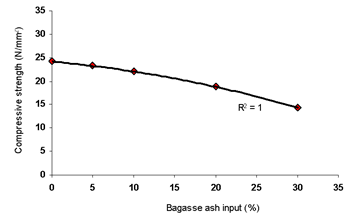• Figure 4. The coefficient of determination between the compressive strength of concrete and bagasse ash input as predicted by derived model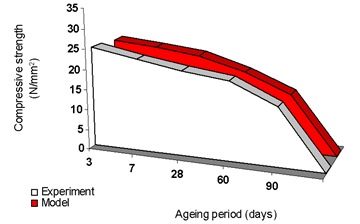• Figure 5. The comparison of compressive strength values of concrete (relative to its ageing period) as obtained from both experiment and derived model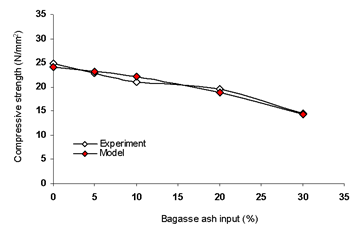• Figure 6. The comparison of compressive strength values of concrete (relative to bagasse ash input) as obtained from both experiment and derived model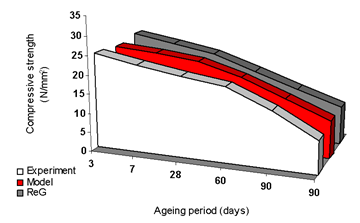• Figure 7. The comparison of compressive strength values of concrete (relative to its ageing period) as obtained from experiment , derived and regression models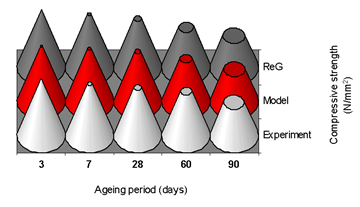• Figure 8. The comparison of compressive strength values of concrete (relative to its ageing period) as obtained from experiment , derived and regression models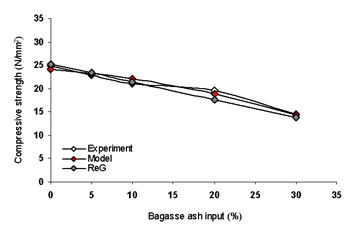• Figure 9. The comparison of compressive strength values of concrete (relative to bagasse ash input) as obtained from experiment , derived and regression models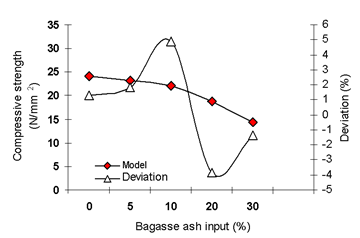• Figure 10. The variation of compressive strength with bagasse ash input and associated deviation (of model-predicted values)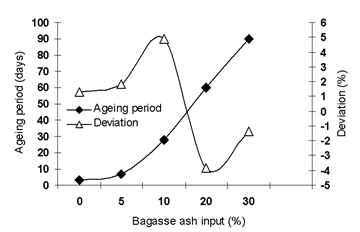• Figure 11. The variation of ageing periods with bagasse ash input and associated deviation (of model-predicted values)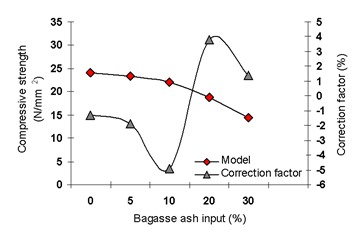• Figure 12. The variation of compressive strength with bagasse ash input and associated correction factor to model-predicted values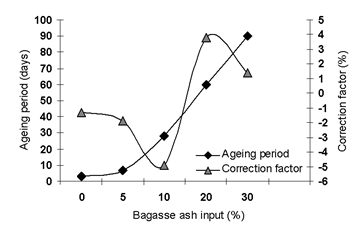• Figure 13. The variation of ageing period with bagasse ash input and associated correction factor to model-predicted values